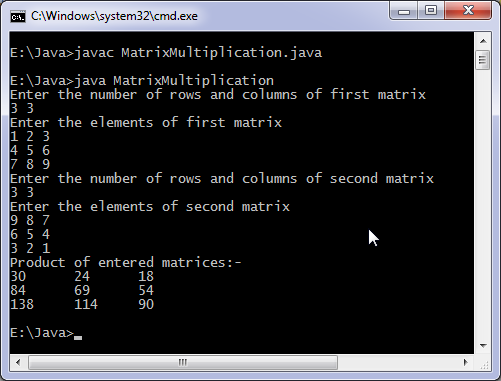# Java program to multiply two matrices

Levels of difficulty: / perform operation:

This java program multiply two matrices. Before multiplication matrices are checked whether they can be multiplied or not.

## This java program

```import java.util.Scanner;
class MatrixMultiplication {
public static void main(String args[]) {
int m, n, p, q, sum = 0, c, d, k;
Scanner in = new Scanner(System.in);
System.out.println("Enter the number of rows and columns of first matrix");
m = in.nextInt();
n = in.nextInt();
int first[][] = new int[m][n];
System.out.println("Enter the elements of first matrix");
for ( c = 0 ; c < m ; c++ )
for ( d = 0 ; d < n ; d++ )
first[c][d] = in.nextInt();
System.out.println("Enter the number of rows and columns of second matrix");
p = in.nextInt();
q = in.nextInt();
if ( n != p )
System.out.println("Matrices with entered orders can't be multiplied ");
else {
int second[][] = new int[p][q];
int multiply[][] = new int[m][q];
System.out.println("Enter the elements of second matrix");
for ( c = 0 ; c < p ; c++ )
for ( d = 0 ; d < q ; d++ )
second[c][d] = in.nextInt();
for ( c = 0 ; c < m ; c++ ) {
for ( d = 0 ; d < q ; d++ ) {
for ( k = 0 ; k < p ; k++ ) {
sum = sum + first[c][k]*second[k][d];
}
multiply[c][d] = sum;
sum = 0;
}
}
System.out.println("Product of entered matrices:-");
for ( c = 0 ; c < m ; c++ ) {
for ( d = 0 ; d < q ; d++ )
System.out.print(multiply[c][d]+"\t");
System.out.print("\n");
}
}
}
}
```

### OutputThis is a basic method of multiplication, there are more efficient algorithms available. Also this approach is not recommended for sparse matrices which contains a large number of elements as zero.